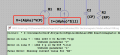# Help to identify a component in the LTSpice.

#### Papabravo

Joined Feb 24, 2006
20,394
It looks like a symbol that is common in control systems where you compute the difference between a setpoint and the feedback to produce the error signal. IMHO it is measuring the voltage across the capacitor and calling it "displacement" which is the mechanical analog in a 2nd order differential equation of the standard RLC circuit.

#### Nick Long

Joined May 12, 2020
75
It looks like a symbol that is common in control systems where you compute the difference between a setpoint and the feedback to produce the error signal. IMHO it is measuring the voltage across the capacitor and calling it "displacement" which is the mechanical analog in a 2nd order differential equation of the standard RLC circuit.
Do you know how to find it in the LTSpice? Thanks.

#### Papabravo

Joined Feb 24, 2006
20,394
Do you know how to find it in the LTSpice? Thanks.
No - I don't. Try asking the author of the Ltspice schematic.
EDIT: Here is the one from the LTspice Control LIbrary available from @Bordodynov or Github. It would be a trivial task to modify the symbol and point to the existing implementation of the function.

httP://Bordodynov.ltwiki.org

Last edited:

#### Nick Long

Joined May 12, 2020
75
No - I don't. Try asking the author of the Ltspice schematic.
EDIT: Here is the one from the LTspice Control LIbrary available from @Bordodynov or Github. It would be a trivial task to modify the symbol and point to the existing implementation of the function.

httP://Bordodynov.ltwiki.org
Thanks. I think the component you recommend has the same function. I have tried it.

#### Nick Long

Joined May 12, 2020
75
No - I don't. Try asking the author of the Ltspice schematic.
EDIT: Here is the one from the LTspice Control LIbrary available from @Bordodynov or Github. It would be a trivial task to modify the symbol and point to the existing implementation of the function.

httP://Bordodynov.ltwiki.org
Sorry, I got another problem. Do you know how to obtain the voltage and current using the function?